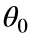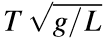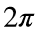# Problem 54710. Compute the period of a pendulum started from a finite initial angle

Cody Problem 49830 asks for the period T of a pendulum swinging through a small angle. Here the pendulum started at rest from an anglethat is not necessarily small. The other assumptions are similar (no friction or drag, massless rod).
Write a function that takes the initial angle and returns, where L is the length of the pendulum and g is the acceleration of gravity. In the limit as the initial angle approaches zero, the function should produce.

### Solution Stats

5.56% Correct | 94.44% Incorrect
Last Solution submitted on Jul 07, 2022

### Community Treasure Hunt

Find the treasures in MATLAB Central and discover how the community can help you!

Start Hunting!